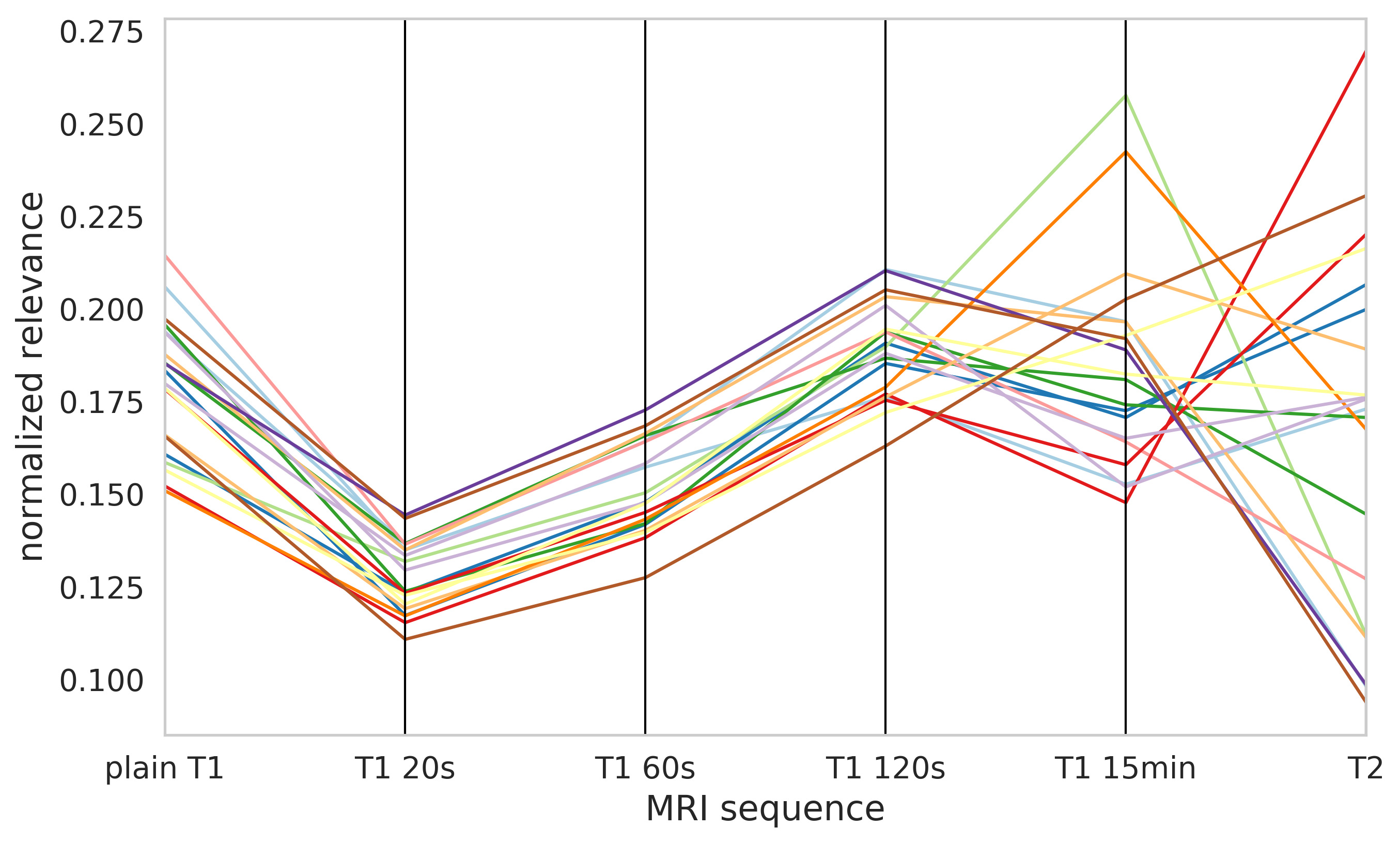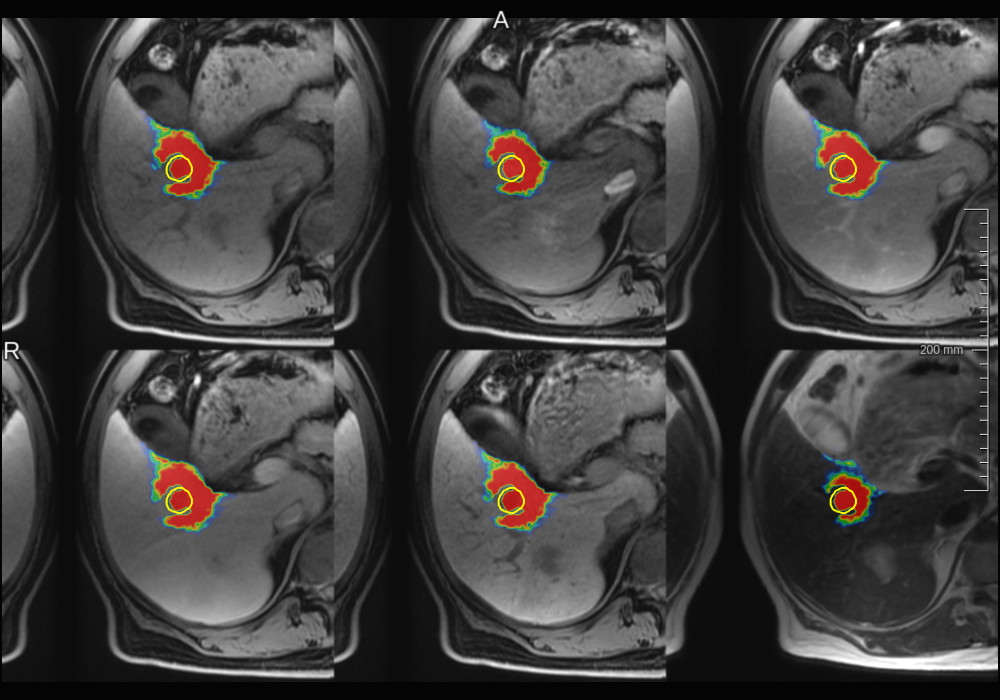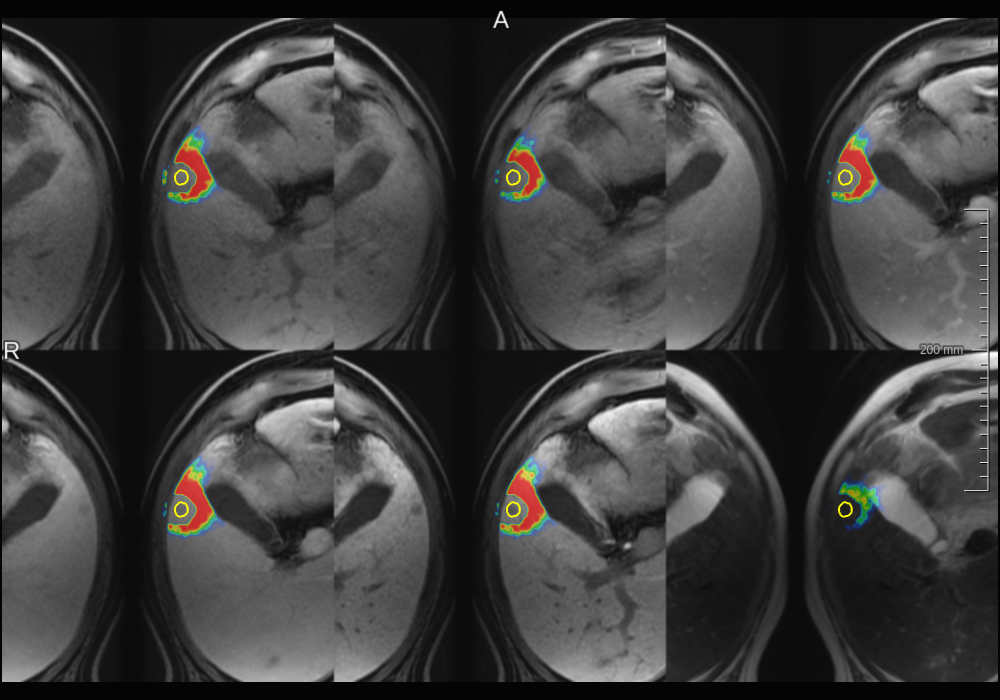### Layer-wise relevance propagation

Layer-wise relevance propagation allows to obtain a pixel-wise decomposition of a~model decision and is applicable for most state-of-the-art architectures for image classification and neural reinforcement learning1. LRP uses a notion of relevance $$R$$ which is equal to the model output for the output neurons and can be propagated to lower layers.

#### LRP for image classification

LRP can be employed to explain classification decisions for a given class $$i$$ by relevance propagation from the corresponding model output $y^i$ according to:

$y^i = R = \ldots = \sum_{d \in L^l} R_d^{(l)} = \ldots = \sum_{d \in L^1} R_d^{(1)} = \sum M^i \ldots (1)$

where $$l$$ refers to the layer index, $$L^l$$ to all neurons of layer $$l$$, and $$R_d^{(l)}$$ to a relevance of neuron $$d$$ in layer $$l$$. Typically, the relevances are propagated to the input layer ($$l=1$$) yielding a relevance map $$M^i$$, which enables visualization of input regions influencing the model decision.

#### LRP for semantic segmentation

In order to apply LRP to semantic segmentation models, we cast the segmentation problem as a voxel-wise classification. This means that in order to explain a decision of a segmentation model for a given output region $$A$$, we propose to compute the input relevance maps according to Eq.1 for each considered output location $$a \in A$$. Then the relevance map $$M^i$$ explaining the model decision for class $$i$$ in the region $$A$$ can be calculated as:

$M^i_A = \sum_{a \in A} \frac{M_a^i}{\sum M_a^i}$

We normalize $$M_a^i$$ by its sum to ensure that each output location $$a$$ equally contributes to the final relevance map $$M^i$$.

### Relevance analysis of liver tumor segmentation CNN

We train a 3D u-net2 with a 6-channel input and 2-channel output to segment liver tumors in : T2, non contrast enhanced T1 (plain-T1), and four dynamic contrast enhanced (DCE) T1 images acquired 20s (T1-20s), 60s (T1-60s), 120s (T1-120s), and 15min (T1-15min) after contrast agent administration (Gd-EOB-DTPA). All sequences were motion corrected using the T1-15min image as reference3.

#### MRI sequence relevance

Normalized relevance distribution across input MRI sequences for 20 test patients denoted by different colors.#### Pixel-level explanations

Foreground relevance maps computed for a true positive.Foreground relevance maps computed for a false negative.#### Discussion

• CNN used information from all MRI sequences.
• T1-15min sequence, which was used to create training labels was not the most important one.
• Similar relevance attribution for plain T1, T1-20s, T1-60s, and T1-120s.
• Pixel-level explanations are hard to interpret.
1. S. Bach et al., On pixel-wise explanations for non-linear classifier decisions by layer-wise relevance propagation. PLOS ONE, 2015.

2. Ö. Çiçek et al. 3d u-net: learning dense volumetric segmentation from sparse annotation. MICCAI, 2016.

3. J. Strehlow et al. Landmark-based evaluation of a deformable motion correction for dce-mri of the liver. IJCARS, 2018.